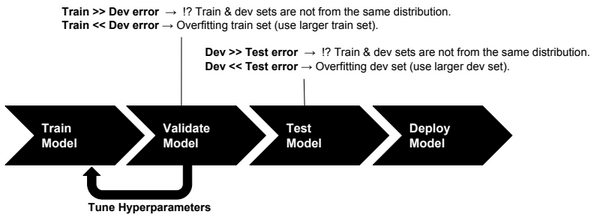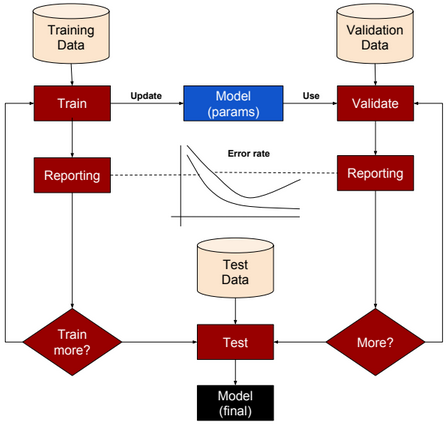# Large/Small Neural Networks

For a large amount of training data, use large networks.

# Applying Machine Learning

Below the steps to apply machine learning to a business problem.

1-Collect DataSet

2-Prepare DataSet

3-Select & Design Model

4-Train Model

5-Evaluate Model

6-Test Model

# Train/Dev/Test SetsTraining set: a set of examples used for training a ML model.

Dev (Validation or Cross-Validation) set: a set of examples used to tune hyper-parameters of a ML model.

Test set: a set of examples used to evaluate how well the model does with data outside the training/Dev set.

For deep learning algorithms, we no longer need to follow the common rules of (70%, 30%) ratios for Train and Test sets or (70%, 15%, 15%) ratios for Train, Dev and Test sets. Splitting data into 98% as training set and 2% as test set could be an acceptable option.

# Error Analysis

Let us define first the following acronyms:

TP: True positive

FP: False positive

TN: True negative

FN: False negative

 Accuracy (TP + TN) / (TP + TN + FP + FN) Precision TP / (TP + FP) Recall TP / (TP + FN) F1 score (best error metric) 2 (Precision * Recall) /(Precision + Recall)

Accuracy alone is a bad measure when data is skewed.